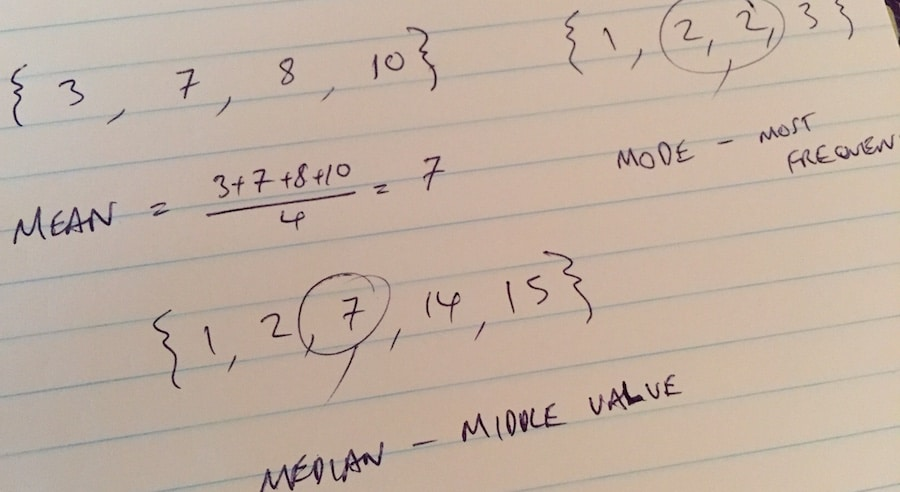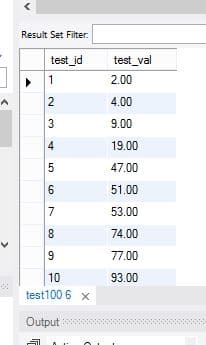# Hang on a Median, whaddya Mean there’s no Mode function in MySQL?Calculating median and mode values for a dataset in MySQL is more complicated than you might expect.

Sure, there is the avg function to calculate mean, which is simple to use, but what about finding the median or mode average values? There are no equivalent functions in MySQL, so we have to work a bit harder to compute these values.

There are many ways to do this. Below I show the approach I take to calculate mean, median and mode values, along with sharing some resources at the end of this post that continue the discussion on the merits of different approaches.

## Setting up the test data

Let’s create some test data – a simple table, called `test100`, consisting of 100 numbers that we want to calculate averages for – by running this query in MySQL:

```create table test100 (
test_id  int(5)  primary key auto_increment,
test_val decimal(9,2)
);

insert into test100 (test_val)
values (2),(4),(9),(19),(47),(51),(53),(74),(77),(93),
(121),(127),(130),(136),(137),(138),(170),(178),(178),(190),
(195),(200),(210),(237),(239),(248),(249),(253),(259),(262),
(264),(264),(278),(286),(296),(327),(327),(328),(329),(329),
(333),(339),(346),(361),(367),(375),(381),(385),(398),(434),
(444),(452),(462),(469),(469),(488),(492),(497),(499),(519),
(539),(557),(560),(560),(578),(593),(601),(609),(622),(638),
(645),(650),(663),(670),(681),(682),(721),(730),(743),(756),
(764),(784),(786),(796),(797),(815),(823),(842),(873),(882),
(885),(888),(915),(920),(935),(938),(956),(960),(962),(975);
```

This gives us a test data table in MySQL as follows:Test 100 table

## Calculation of the Mean value

The mean is the “average” that we’re all familiar with, the one we usually refer to when we say “average”, and it’s calculated as the sum of the values divided by the count of the values.

The calculation is easily handled in MySQL using the `avg` function , as follows:

```select avg(test_val)
from test100
```

To get the answer to 2 decimal places, I can wrap a format function around the avg function, as shown below. In this example, I’ve called the column “mean” using the as statement.

```select format(avg(test_val),2) as mean
from test100
```

Note, we can also calculate the mean value using the `sum` and `count` functions, which gives the same result as the `avg` function:

```select format(sum(test_val)/count(test_val),2) as mean
from test100
```

For our test dataset, the mean is: 461.18

## Calculation of the Median value

Median is the middle value, the numerical value that separates the higher half of the data from the lower half.

E.g. consider the dataset `{ 1, 2, 3 }` with an odd number of values, then the median value is the middle value, in this case 2

Consider a dataset `{ 1, 2, 3, 4 }` with an even number of values, where the median is then an average (mean) of the middle two values, namely `(2 + 3)/2`, which is 2.5

The steps to create a query to do this in MySQL are:

Step 1: create an intermediary table where the data is sorted and numbered.

```select @counter:=@counter+1 as 'Row', test_val
from test100, (select @counter:=0) r
order by 2
```

This creates a table with the data sorted from smallest to largest with each row numbered from 1 to 100. We created a variable (called `counter`) and then incremented this by 1 for each row. The final line of the query orders the data by column 2, i.e. the test value.

Step 2: The second table we need for the median calculation is a simple count to determine the total number of rows of data in our dataset.

```select count(*) as count
from test100
```

Step 3: as a baby next step, let’s calculate the median for the specific case of our test data, where we have exactly 100 values in the dataset. Note, this scenario calculates the median for an even number of rows, by finding the middle two rows and taking the mean value.

Here, I order the data as per step 1 (inner subquery) and then I take the average of rows 50 and 51, to calculate the median (see lines 1 and 7, the outer wrapper).

```select avg(test_val) as median from
(
select @counter:=@counter+1 as row_id, t1.test_val
from test100 t1, (select @counter:=0) t2
order by t1.test_val
) t
where t.row_id in (50, 51)
```

Step 4: adjust calculation to account for any number of table rows.

So far, we’ve looked specifically at 100 rows of data, i.e. an even number of values. Recall, it’s simpler for a dataset with an odd number of values: then it’s simply the middle value.

We modify the preceding query in step 3 to be more general by replacing the `(50, 51)` set with a subquery to determine the total number of rows and then a calculation to find the middle values.

We use the table from step 2 to calculate the total number of rows of data.

Then we specify that our `row_id` must be the middle value (odd number of values) or the two middle values (even number of values), using the `floor()` MySQL function, which returns the largest integer not greater than the argument.

Use the formula:

`(floor((total_rows + 1)/2), floor((total_rows + 2)/2))`

to find the middle value or values of our dataset.

Let’s look at a super simple example to see how this calculation works:

Case 1: an odd number set e.g. { 1, 2, 3 }

• `total_rows = 3`
• ```(floor((total_rows + 1)/2), floor((total_rows + 2)/2)) = (floor((3 + 1)/2), floor((3 + 2)/2)) = (floor(2), floor(2.5)) = (2, 2)```
• So we select rows with a row_id in `( 2, 2 )` i.e. the 2nd row, or middle value

Case 2: an even number set e.g. { 1, 2, 3, 4 }

• `total_rows = 4`
• ```(floor((total_rows + 1)/2), floor((total_rows + 2)/2)) = (floor((4 + 1)/2), floor((4 + 2)/2)) = (floor(2.5), floor(3)) = (2, 3)```
• So we select rows with a row_id in `( 2, 3 )` i.e. the 2nd and 3rd rows
• We then take an average (using mean) of the values in the 2nd and 3rd rows to get the median

In MySQL, our query now looks like this:

```select avg(test_val) as median from
(
select @counter:=@counter+1 as row_id, t1.test_val
from test100 t1, (select @counter:=0) t2
order by t1.test_val
) o1
join
(
select count(*) as total_rows
from test100
) o2
where o1.row_id in (floor((o2.total_rows + 1)/2), floor((o2.total_rows + 2)/2))
```

For our test dataset, the median is: 439.00

## Calculation of the Mode value

The mode is the other average value, representing the most frequent value in a dataset.

This is achieved in MySQL using the `count` function to identify the most frequent values in the dataset.

Step 1: count the frequencies of values in the dataset, by grouping on the value and using the count function.

```select test_id, test_val, count(test_val) as max_count from test100
group by test_val
```

Note, we could order by frequency at this stage, and then manually read off the mode value(s) and call it a day:

```select test_id, test_val, count(test_val) as max_count from test100
group by test_val
order by max_count desc
```

However, let’s continue and flesh out a full query solution.

Step 2: select the max frequency value from our table of values and frequencies.

```select max(count_val) from
(
select test_id, test_val, count(test_val) as count_val from test100
group by test_val
) t
```

Step 3: setup the unfiltered query.

```select test_id, test_val, max_count
from
(
select test_id, test_val, count(test_val) as max_count from test100
group by test_val
) t1
```

Step 4: add the query to filter on max_count, by specifying a `where` clause so that only values with a frequency count in the max count table (from step 2) are returned.

```select test_id, test_val, max_count
from
(
select test_id, test_val, count(test_val) as max_count from test100
group by test_val
) t1
where max_count in
(
select max(count_val) from
(
select test_id, test_val, count(test_val) as count_val from test100
group by test_val
) t
)
```

Step 5: add a condition on max_count to ensure it is greater than 1, so we only return valid results when the mode exists (no mode if all the values occur only once).

```select test_id, test_val, max_count
from
(
select test_id, test_val, count(test_val) as max_count from test100
group by test_val
) t1
where max_count in
(
select max(count_val) from
(
select test_id, test_val, count(test_val) as count_val from test100
group by test_val
) t
)
and max_count > 1```

For our test dataset, there are 6 mode values: 178, 264, 327, 329, 469, 560

And that’s it. As you can see, it’s not entirely straightforward to calculate the median and mode values in MySQL, but it it’s not rocket science either. Hopefully this post will serve as an introduction to performing these calculations in MySQL.

### Further reading

Further reading in these Stack Overflow and blog posts: